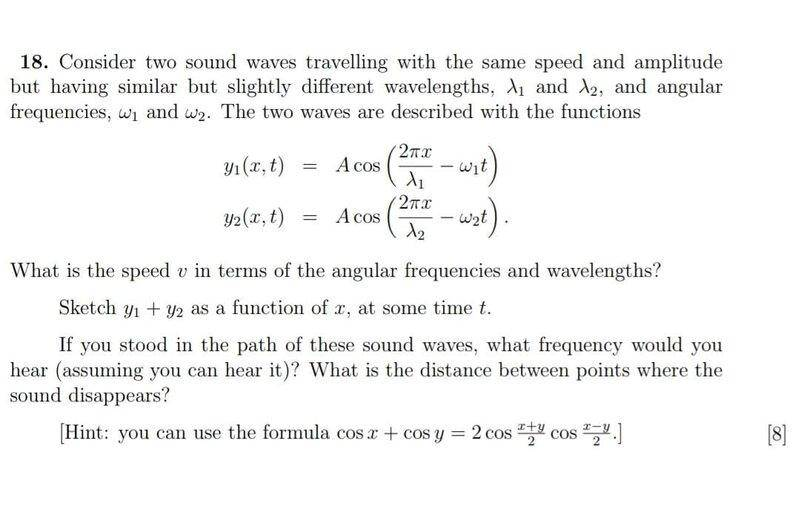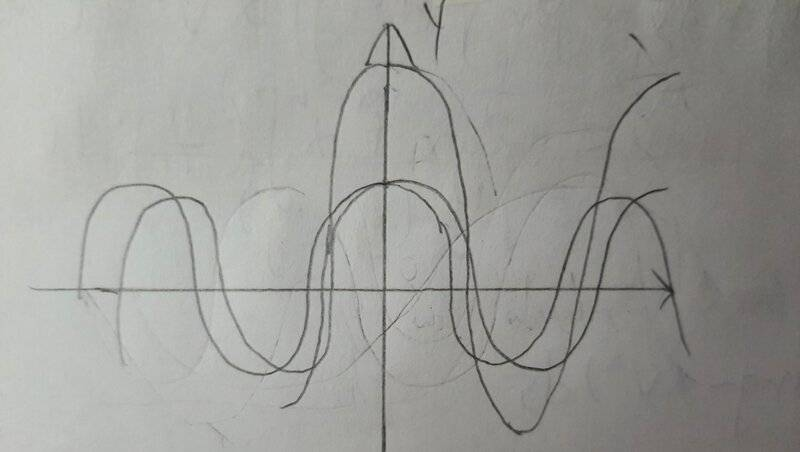# Resultant Frequency and Wavelength of Interfering Sound Waves

• Codemetry
In summary, the conversation discusses the use of angular frequency and linear frequency in equations describing waves. It also mentions the relationship between these two frequencies and how they can be used interchangeably. The question at hand involves finding the speed v in terms of angular frequencies and wavelengths, and drawing a graph of y1+y2 as a function of x. The conversation also addresses the possibility of solving this question with the knowledge of A-level physics, mentioning that it is suitable for students familiar with handling sines and cosines. Finally, the question is asked how an angular frequency can have a wavelength, and the conversation clarifies that despite its name, angular frequency can be applied to non-rotating things.f

#### Codemetry

Homework Statement
What is the speed ##v## in terms of the angular frequencies and wavelengths?
Sketch ##y1+y2## as a function of ##x##, at some time ##t##.
If you stood in a path of these sound waves, what frequency would you hear? What is the distance between points where the sound disappears?
Relevant Equations
$$v=f\lambda$$
$$y(x,t)=Acos(\frac{2\pi x}{\lambda}-\omega t)$$##-w1## and ##-w2## are to shift the cosine graph to the right, and ##\frac{2pi}{\lambda}## is to stretch the graph. But I can't seem to draw an appropriate ##y1+y2## graph (quite irregular) and I struggle to find the resultant frequency and wavelength. Also, why is there angular frequency in a sound wave?
Is it possible to solve this question with the knowledge of A-level physics? (##v=f\lambda##, transverse wave, longitudinal wave, etc.)

Last edited:
Homework Statement:: What is the speed v in terms of the angular frequencies and wavelengths?
Sketch y1+y2 as a function of x, at some time t.
If you stood in a path of these sound waves, what frequency would you hear? What is the distance between points where the sound disappears?
Hi @Codemetry. The rules here (https://www.physicsforums.com/threads/homework-help-guidelines-for-students-and-helpers.686781/) require that you first make an attempt before we offer guidance. So...

Have you found v in terms of angular frequencies and wavelengths (the first question)? What do you get?
Have you applied the 'Hint' given in the question? What do you get for y₁ + y₂?

Also, why is there angular frequency in a sound wave?
Angular frequency is often used in equations describing waves.

Angular frequency is usually represented by 'ω' and is typically measured in units of radians per second (rad/s). It is best understood by imagining each cycle corresponds to a point moving one around a circle and then asking 'what angle (in radians) is covered each second?'.

Linear frequency (usually just called 'frequency') is usually represented by 'f' and is typically measured in units of hertz (Hz) which means the same as cycles per second.

You can use ω or f, whichever is most convenient for any type of oscillation or wave because they are simply related by:

##\omega = 2 \pi f## and ##f = \frac{\omega}{2\pi}##
Also note that if T is the period, then ##f = \frac {1}{T}## and ##\omega = \frac {2\pi}{T}##.

If you are not familiar with these relationships, check your notes/textbook.
Is it possible to solve this question with the knowledge of A-level physics? (v=f*lambda, transverse wave, longitudinal wave, etc.)
The question is suitable for A-level physics students assuming they are familiar with handling sines and cosines (e.g. using the rule provided in the hint in the question). If you have not covered this in A-level maths, you may have to do some extra reading about sines and cosines.

Hi @Codemetry. The rules here (https://www.physicsforums.com/threads/homework-help-guidelines-for-students-and-helpers.686781/) require that you first make an attempt before we offer guidance. So...

Have you found v in terms of angular frequencies and wavelengths (the first question)? What do you get?
Have you applied the 'Hint' given in the question? What do you get for y₁ + y₂?

Angular frequency is often used in equations describing waves.

Angular frequency is usually represented by 'ω' and is typically measured in units of radians per second (rad/s). It is best understood by imagining each cycle corresponds to a point moving one around a circle and then asking 'what angle (in radians) is covered each second?'.

Linear frequency (usually just called 'frequency') is usually represented by 'f' and is typically measured in units of hertz (Hz) which means the same as cycles per second.

You can use ω or f, whichever is most convenient for any type of oscillation or wave because they are simply related by:

##\omega = 2 \pi f## and ##f = \frac{\omega}{2\pi}##
Also note that if T is the period, then ##f = \frac {1}{T}## and ##\omega = \frac {2\pi}{T}##.

If you are not familiar with these relationships, check your notes/textbook.

The question is suitable for A-level physics students assuming they are familiar with handling sines and cosines (e.g. using the rule provided in the hint in the question). If you have not covered this in A-level maths, you may have to do some extra reading about sines and cosines.
How does an angular frequency have a wavelength? (As opposed to linear frequencies where wavelength is the distance between two crests)
Like I said I got an irregular graph for y1+y2 (by plotting y1 and y2 and visually adding them together, since it is quite impossible to plot a graph using the formula derived with the hint)

Have you found v in terms of angular frequencies and wavelengths (the first question)? What do you get?
Have you applied the 'Hint' given in the question? If so] What do you get for y₁ + y₂?

In the meantime, note that despite the name, angular frequency can be applied to things that are not rotating. For example a sound wave with ##f=50##Hz has an angular frequency of ##\omega =314## rad/s approx. (because ##\omega = 2\pi f##). Saying ##f=50##Hz is the same as saying ##\omega = 314##rad/s.

•Delta2

Have you found v in terms of angular frequencies and wavelengths (the first question)? What do you get?
Have you applied the 'Hint' given in the question? If so] What do you get for y₁ + y₂?

In the meantime, note that despite the name, angular frequency can be applied to things that are not rotating. For example a sound wave with ##f=50##Hz has an angular frequency of ##\omega =314## rad/s approx. (because ##\omega = 2\pi f##). Saying ##f=50##Hz is the same as saying ##\omega = 314##rad/s.
##\omega=2\pi f## and ##v=f\lambda##, so ##v_1=\frac{\omega_1\lambda_1}{2\pi}## and ##v_2=\frac{\omega_2\lambda_2}{2\pi}##.
$$y_1(x,t)+y_2(x,t)=A(\cos(\frac{2\pi x}{\lambda_1}-\omega_1t)+\cos(\frac{2\pi x}{\lambda_2}-\omega_2t))$$
$$=A\cos(\frac{1}{2}(\frac{2\pi x}{\lambda_1}-\omega_1t+\frac{2\pi x}{\lambda_2}-\omega_2t))\cos(\frac{1}{2}(\frac{2\pi x}{\lambda_1}-\omega_1t-\frac{2\pi x}{\lambda_2}+\omega_2t))$$
$$=A\cos(\pi x(\frac{1}{\lambda_1}+\frac{1}{\lambda_2})-\frac{\omega_1+\omega_2}{2}t)\cos(\pi x(\frac{1}{\lambda_1}-\frac{1}{\lambda_2})-\frac{\omega_1t-\omega_2}{2}t)$$
Applying the hint gives a product of cosines which I struggle to plot a graph for it. That was why I went for plotting ##y_1## and ##y_2## separately then add them visually, but I couldn't plot a significant graph. Also, I don't want to use Desmos.Last edited:
•Steve4Physics
$$=A\cos(\pi x(\frac{1}{\lambda_1}+\frac{1}{\lambda_2})-\frac{\omega_1+\omega_2}{2}t)\cos(\pi x(\frac{1}{\lambda_1}-\frac{1}{\lambda_2})-\frac{\omega_1t-\omega_2}{2}t)$$
Well done. Especially for using Latex so well.

Don’t forget the leading factor ‘2’ in the identity:
##cos(x) + cos(y) = 2cos(\frac {x+y}{2})cos(\frac {x-y}{2})##

And you have written ##\omega_1 t## instead of just ##\omega _1## near the end of the last equation.

We can tidy up your last equation by defining some new variables.
##k_A= \pi (\frac {1}{λ_1} +\frac {1}{λ_2})##
##k_B = \pi (\frac{1}{λ_1} -\frac {1}{λ_2})##
##ω_A = \frac {ω_1 + ω_2}{2}##
##ω_B = \frac {ω_1 – ω_2}{2}##

We end up with
##y_1(x,t)+y_2(x,t)= 2A\cos(k_A x - \omega_A t) \cos(k_B x - \omega_B t)##

We are asked to sketch the graph for ##y_1 +y_2## as a function of x at some time t. Since we are not told the value of t, we are justified in taking t=0. (A different, non-zero, value of t will give the same shape graph but shifted left/right.)

So we need to sketch the graph ##y = 2A\cos(k_Ax) \cos(k_B x) ##

We are told ## λ_1## and ##λ_2## are similar, so k_B is large compared to k_A. (I think it’s that way around!)

To get the general shape we could plot a graph of (for instance) ##y = cos(x)cos(10x)##.

Although it’s now manageable, plotting this by hand is still pretty hard work. I wouldn’t want to do it. Can’t a graphical calculator be used?

If your maths is good (and especially if you are familiar with the maths of ‘beats’) then you might recognise that you have a short wavelength sine-wave amplitude-modulated by a much longer wavelength sine-wave. Then it’s easy to sketch the general shape of the graph.